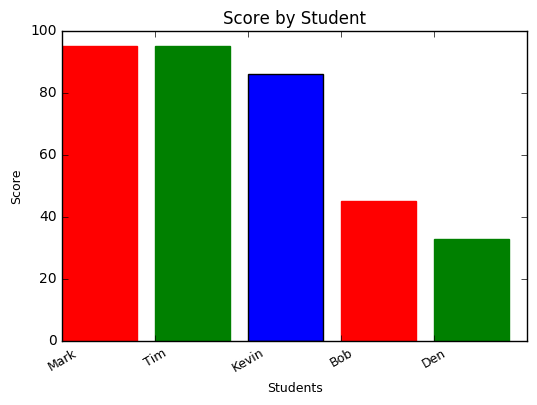Pandas Numpy and Matplotlib

I have created demo code with Jupyter Notebook, which can be viewed here: https://github.com/marcuspaget/pythonDSFromScratch/blob/master/PandasDemo.md

Panda – Python Data Analysis Library

Quick install with:

pip install pandas

Python’s answer to R’s DataFrames for data manipulation

Providing tools to read and write data between data structures and different formats: CSV and text files, Microsoft Excel, SQL databases, and the fast HDF5 format;

Easily to manipulate, slice and dice data, with integrated indexing.

Possible to convert HDF5 to HDFS for ingestion in Hadoop

Time series-functionality:

• Date range generation & modification
• Frequency conversion
• Moving window statistics
• Join time series without losing data

Numpy – Python Number Library

pip install numpy
Create, manipulate , slice and run ops i.e std, mean, min, max, etc

Matplotlib – Python plotting and figures

pip install matplotlib
Graph data from lists, dataframes, etc.

For example:I have created demo code with Jupyter Notebook, which can be viewed here: https://github.com/marcuspaget/pythonDSFromScratch/blob/master/PandasDemo.md

Python Dict

Sample code for working with Python Dicts

# init users list of dicts, print out id 1, then init friends list of tuples

users = [

{ "id": 0, "name": "Bob" },
{ "id": 1, "name": "Dunn" },
{ "id": 2, "name": "Sue" },
{ "id": 3, "name": "Chi" },
{ "id": 4, "name": "Thor" },
{ "id": 5, "name": "Clive" },
{ "id": 6, "name": "Hicks" },
{ "id": 7, "name": "Devin" },
{ "id": 8, "name": "Kate" },
{ "id": 9, "name": "Klein" },
{ "id": 10, "name": "Jen" }

]

i=0
for user in users:
if(users[i]["name"]=="Bob"):
print("Bob ID: ",users[i]["id"])
i+=1

friends= [(0, 1), (0, 2), (1, 2), (1, 3), (2, 3), (3, 4), (4, 5), (5, 6), (5, 7), (6, 8), (7, 8), (8, 9)]

# spin through all users and create empty list to store list, then populate

for user in users:
user["friends"]=[]

# populate empty list with all left side of tuple with right and vice versa

for i,j in friends:
users[i]["friends"].append(users[j])
users[j]["friends"].append(users[i])

# function to return length based on passed in user

def number_of_friends(user):
"""how many friends does _user_ have?"""
return len(user["friends"]) # length of friend_ids list

# total up all friends

total_connection = sum(number_of_friends(user)
for user in users) # 24

# grab number of users

num_users = len(users)

avg_connections = total_connection / num_users # 2.4

# create a list (user_id, number_of_friends)

num_friends_by_id = [(user["id"], number_of_friends(user)) for user in users]

print(sorted(num_friends_by_id,key=lambda pair: pair, reverse=True))

# Output – largest to smallest

[(1, 3), (2, 3), (3, 3), (5, 3), (8, 3), (0, 2), (4, 2), (6, 2), (7, 2), (9, 1), (10, 0)]